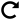# Polar to Rectangular Calculator

Calculate Polar to Rectangular
Enter the Polar Coordinates
r coordinate =

Î¸ coordinate =x-coordinate =

y-coordinate =

Polar to Rectangular Calculator is a free online tool that displays the conversion of polar coordinate to the rectangular coordinate. CoolGyan online polar to rectangular calculator tool makes the calculation faster and it displays the conversion in a fraction of seconds.

## How to Use the Polar to Rectangular Calculator?

The procedure to use polar to rectangular calculator is as follows:

Step 1: Enter the polar coordinate values in the respective input field

Step 2: Now click the button ”Calculate Rectangular Coordinates” to get the result

Step 3: Finally, the conversion of polar to rectangular coordinate will be displayed in the output field

### What is Meant by Polar to Rectangular Coordinate?

In Mathematics, polar to rectangular coordinates represents the conversion of polar to the rectangular coordinates. The rectangular coordinates are called the cartesian coordinate which is of the form (x, y), whereas the polar coordinate is in the form of (r, Î¸). The conversion of polar coordinate to the rectangular coordinate formula is given as

x = r cos Î¸

y = r sin Î¸

Substitute the value of r and Î¸ in the conversion formula, and solve for the x and y value to get the rectangular coordinates.